Updating search results...

# 51 Results

View
Selected filters:
• CCSS.Math.Content.7.RP.A.1Only Sharing Permitted
CC BY-NC-ND
Rating
5.0 stars

This lesson unit is intended to help you assess whether students recognize relationships of direct proportion and how well they solve problems that involve proportional reasoning. In particular, it is intended to help you identify those students who: use inappropriate additive strategies in scaling problems, which have a multiplicative structure; rely on piecemeal and inefficient strategies such as doubling, halving, and decomposition, and have not developed a single multiplier strategy for solving proportionality problems; and see multiplication as making numbers bigger, and division as making numbers smaller.

Subject:
Numbers and Operations
Material Type:
Assessment
Lesson Plan
Provider:
Shell Center for Mathematical Education
Provider Set:
Mathematics Assessment Project (MAP)
04/26/2013Only Sharing Permitted
CC BY-NC-ND
Rating
4.0 stars

This lesson unit is intended to help teachers assess how well students are able to interpret percent increase and decrease, and in particular, to identify and help students who have the following difficulties: translating between percents, decimals, and fractions; representing percent increase and decrease as multiplication; and recognizing the relationship between increases and decreases.

Subject:
Ratios and Proportions
Material Type:
Assessment
Lesson Plan
Provider:
Shell Center for Mathematical Education
Provider Set:
Mathematics Assessment Project (MAP)
04/26/2013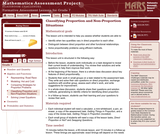Only Sharing Permitted
CC BY-NC-ND
Rating
5.0 stars

This lesson unit is intended to help teachers assess whether students are able to: identify when two quantities vary in direct proportion to each other; distinguish between direct proportion and other functional relationships; and solve proportionality problems using efficient methods.

Subject:
Ratios and Proportions
Material Type:
Assessment
Lesson Plan
Provider:
Shell Center for Mathematical Education
Provider Set:
Mathematics Assessment Project (MAP)
04/26/2013Only Sharing Permitted
CC BY-NC-ND
Rating
4.5 stars

This lesson unit is intended to help you assess how well students are able to: solve simple problems involving ratio and direct proportion; choose an appropriate sampling method; and collect discrete data and record them using a frequency table.

Subject:
Education
Geometry
Measurement and Data
Ratios and Proportions
Material Type:
Assessment
Lecture Notes
Lesson Plan
Teaching/Learning Strategy
Provider:
Shell Center for Mathematical Education
Provider Set:
Mathematics Assessment Project (MAP)
Author:
http://map.mathshell.org/
04/26/2013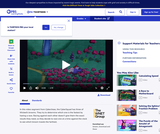Educational Use
Rating
0.0 stars

The CyberSquad tests which broom can travel the furthest in five seconds in this video from Cyberchase.

Subject:
Mathematics
Physics
Material Type:
Lecture
Provider:
PBS LearningMedia
Provider Set:
PBS Learning Media: Multimedia Resources for the Classroom and Professional Development
Author:
U.S. Department of Education
WNET
07/08/2008Only Sharing Permitted
CC BY-NC-ND
Rating
4.5 stars

This lesson unit is intended to help assess how well students are able to interpret and use scale drawings to plan a garden layout. This involves using proportional reasoning and metric units.

Subject:
Algebra
Geometry
Ratios and Proportions
Material Type:
Assessment
Lesson Plan
Provider:
Shell Center for Mathematical Education
Provider Set:
Mathematics Assessment Project (MAP)
04/26/2013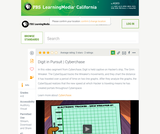Educational Use
Rating
0.0 stars

The CyberSquad tracks Digital position in time and then studies graphs to figure out what Hacker is scheming in this video from Cyberchase.

Subject:
Mathematics
Physics
Material Type:
Lecture
Provider:
PBS LearningMedia
Provider Set:
PBS Learning Media: Multimedia Resources for the Classroom and Professional Development
Author:
U.S. Department of Education
WNET
09/25/2008Rating
4.0 stars

Use the Sound Grapher to create visualizations of sound and learn about the frequency, wavelength, amplitude and velocity of sound waves.

Subject:
Chemistry
Physics
Material Type:
Activity/Lab
Lecture Notes
Provider:
Concord Consortium
Provider Set:
Concord Consortium Collection
Author:
The Concord Consortium
12/11/2011Rating
0.0 stars

Getting Started

Type of Unit: Introduction

Prior Knowledge

Students should be able to:

Understand ratio concepts and use ratios.
Use ratio and rate reasoning to solve real-world problems.
Identify and use the multiplication property of equality.

Lesson Flow

This unit introduces students to the routines that build a successful classroom math community, and it introduces the basic features of the digital course that students will use throughout the year.

An introductory card sort activity matches students with their partner for the week. Then over the course of the week, students learn about the routines of Opening, Work Time, Ways of Thinking, Apply the Learning (some lessons), Summary of the Math, Reflection, and Exercises. Students learn how to present their work to the class, the importance of students’ taking responsibility for their own learning, and how to effectively participate in the classroom math community.

Students then work on Gallery problems, to further explore the resources and tools and to learn how to organize their work.

The mathematical work of the unit focuses on ratios and rates, including card sort activities in which students identify equivalent ratios and match different representations of an equivalent ratio. Students use the multiplication property of equality to justify solutions to real-world ratio problems.

Subject:
Mathematics
Material Type:
Unit of Study
Provider:
PearsonConditional Remix & Share Permitted
CC BY-NC
Rating
0.0 stars

Students write the relationship between two fractions as a unit rate and use unit rates and the constant of proportionality to solve problems involving proportional relationships.Key ConceptsIn situations where there is a constant rate involved, the unit rate is a constant of proportionality between the two variable quantities and can be used to write a formula of the form y = kx.A given constant rate can be simplified to find the unit rate by expressing its value with a denominator of 1.The ratios of two fractions can be expressed as a unit rate.Goals and Learning ObjectivesExpress the ratios of two fractions as a unit rate.Understand that when a constant rate is involved, the unit rate is the constant of proportionality.Use the unit rate to write and solve a formula of the form y = kx.

Subject:
Ratios and Proportions
Material Type:
Lesson Plan
Provider:
Pearson
09/21/2015Conditional Remix & Share Permitted
CC BY-NC
Rating
0.0 stars

Math in Real Life (MiRL) supports the expansion of regional networks to create an environment of innovation in math teaching and learning.  The focus on applied mathematics supports the natural interconnectedness of math to other disciplines while infusing relevance for students.  MiRL supports a limited number of networked math learning communities that focus on developing and testing applied problems in mathematics.  The networks help math teachers refine innovative teaching strategies with the guidance of regional partners and the Oregon Department of Education.

Subject:
Mathematics
Material Type:
Homework/Assignment
Author:
Mark Freed
Tom Thompson
07/27/2020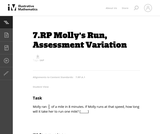Unrestricted Use
CC BY
Rating
0.0 stars

This is a task from the Illustrative Mathematics website that is one part of a complete illustration of the standard to which it is aligned. Each task has at least one solution and some commentary that addresses important asects of the task and its potential use. Here are the first few lines of the commentary for this task: Molly ran $\frac{2}{3}$ of a mile in 8 minutes. If Molly runs at that speed, how long will it take her to run one mile? [_____]...

Subject:
Mathematics
Material Type:
Activity/Lab
Provider:
Illustrative Mathematics
Provider Set:
Illustrative Mathematics
Author:
Illustrative Mathematics
03/18/2013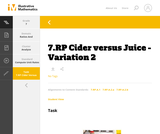Unrestricted Use
CC BY
Rating
0.0 stars

This is a task from the Illustrative Mathematics website that is one part of a complete illustration of the standard to which it is aligned. Each task has at least one solution and some commentary that addresses important aspects of the task and its potential use.

Subject:
Ratios and Proportions
Material Type:
Activity/Lab
Provider:
Illustrative Mathematics
Provider Set:
Illustrative Mathematics
Author:
Illustrative Mathematics
08/06/2015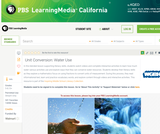Educational Use
Rating
0.0 stars

In this lesson designed to enhance literacy skills, students examine how to use fractions to measure and help conserve freshwater resources.

Subject:
Mathematics
Material Type:
Activity/Lab
Provider:
PBS LearningMedia
Provider Set:
PBS Learning Media Common Core Collection
Author:
Walmart Foundation
WGBH Educational Foundation
08/23/2012Conditional Remix & Share Permitted
CC BY-NC
Rating
0.0 stars

Students analyze the graph of a proportional relationship in order to find the approximate constant of proportionality, to write the related formula, and to create a table of values that lie on the graph.Key ConceptsThe constant of proportionality determines the steepness of the straight-line graph that represents a proportional relationship. The steeper the line is, the greater the constant of proportionality.On the graph of a proportional relationship, the constant of proportionality is the constant ratio of y to x, or the slope of the line.A proportional relationship can be represented in different ways: a ratio table, a graph of a straight line through the origin, or an equation of the form y = kx, where k is the constant of proportionality.Goals and Learning ObjectivesIdentify the constant of proportionality from a graph that represents a proportional relationship.Write a formula for a graph that represents a proportional relationship.Make a table for a graph that represents a proportional relationship.Relate the constant of proportionality to the steepness of a graph that represents a proportional relationship (i.e., the steeper the line is, the greater the constant of proportionality).

Subject:
Ratios and Proportions
Material Type:
Lesson Plan
Provider:
Pearson
09/21/2015Conditional Remix & Share Permitted
CC BY-NC
Rating
0.0 stars

Four full-year digital course, built from the ground up and fully-aligned to the Common Core State Standards, for 7th grade Mathematics. Created using research-based approaches to teaching and learning, the Open Access Common Core Course for Mathematics is designed with student-centered learning in mind, including activities for students to develop valuable 21st century skills and academic mindset.

Subject:
Mathematics
Material Type:
Full Course
Provider:
Pearson
10/06/2016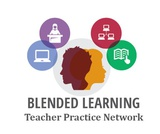Conditional Remix & Share Permitted
CC BY-NC
Rating
0.0 stars

In this problem-based learning module, students will raise awareness about the amount of excess food wasted each day in America’s schools highlights the consumptive mindset that our county has.  Each day in third world countries, as well as our own, children go without the proper nutrition needed to focus on other activities. This module will raise student awareness about the amount of food taken from the service line and then placed in waste receptacles.  Students will gather and analyze data regarding wasted food in an attempt to design a solution to reduce food waste. Sation rotation will allow to conduct research into the global problems associated with the lack of food and malnutrition. Mathematical skills will be required to calculate rates and percentages of consumption which will be used for analysis.

Subject:
English Language Arts
Mathematics
Material Type:
Lesson Plan
Author:
Blended Learning Teacher Practice Network
07/27/2018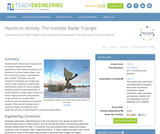Educational Use
Rating
0.0 stars

Students learn about radar imaging and its various military and civilian applications that include recognition and detection of human-made targets, and the monitoring of space, deforestation and oil spills. They learn how the concepts of similarity and scaling are used in radar imaging to create three-dimensional models of various targets. Students apply the critical attributes of similar figures to create scale models of a radar imaging scenario using infrared range sensors (to emulate radar functions) and toy airplanes (to emulate targets). They use technology tools to measure angles and distances, and relate the concept of similar figures to real-world applications.

Subject:
Education
Material Type:
Activity/Lab
Provider:
TeachEngineering
Provider Set:
TeachEngineering
Author:
Mounir Ben Ghalia
Rocio Denise Nava
10/14/2015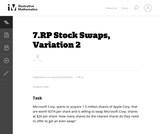Unrestricted Use
CC BY
Rating
0.0 stars

This problem can be solved in more than one way. The choice in solution method may reflect the comfort and mathematical sophistication of the student.

Subject:
Mathematics
Material Type:
Activity/Lab
Provider:
Illustrative Mathematics
Provider Set:
Illustrative Mathematics
Author:
Illustrative Mathematics
05/01/2012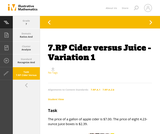Unrestricted Use
CC BY
Rating
0.0 stars

This is a task from the Illustrative Mathematics website that is one part of a complete illustration of the standard to which it is aligned. Each task has at least one solution and some commentary that addresses important aspects of the task and its potential use.

Subject:
Ratios and Proportions
Material Type:
Activity/Lab
Provider:
Illustrative Mathematics
Provider Set:
Illustrative Mathematics
Author:
Illustrative Mathematics